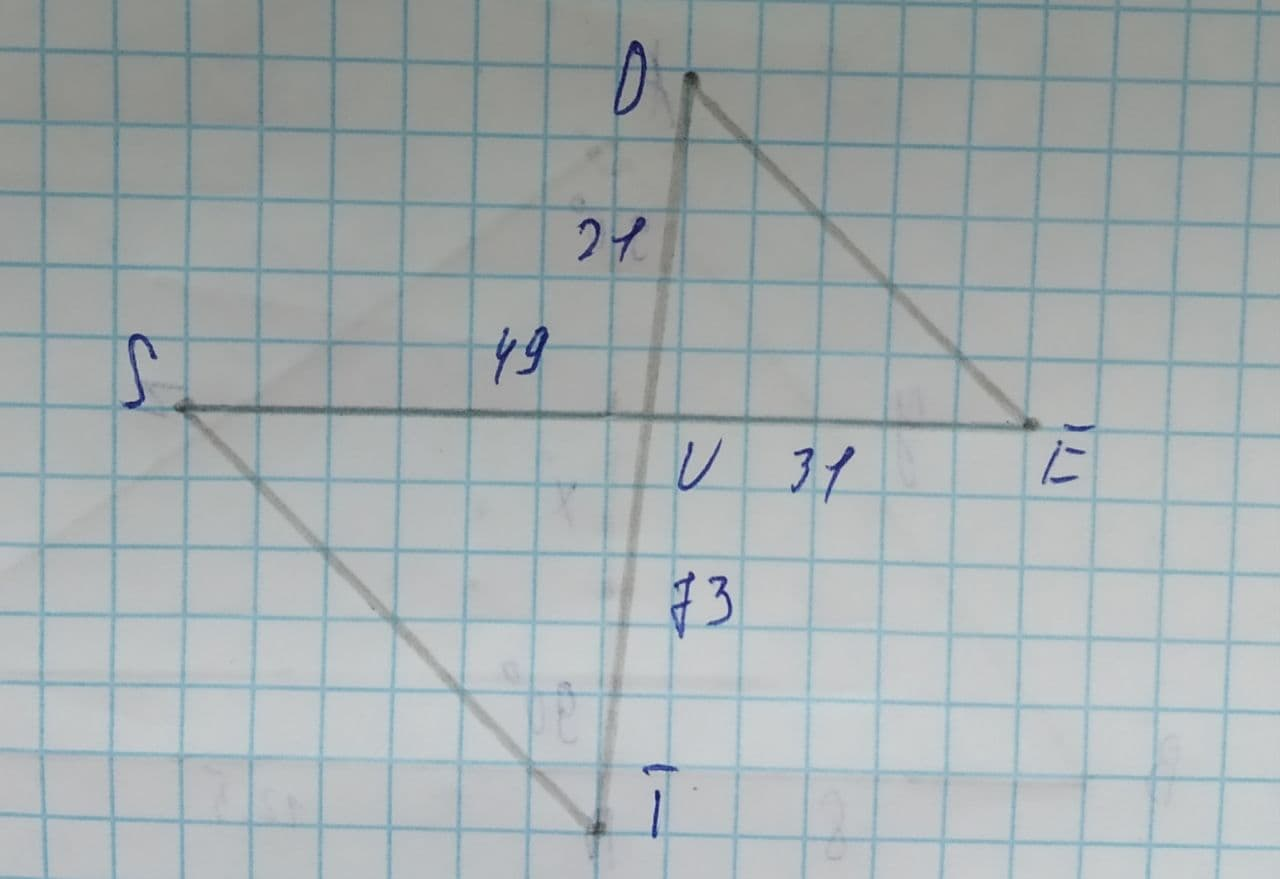# Are the two triangles similar? If so, by what similarity shortcut?Burhan Hopper 2021-08-05 Answered
Are the two triangles similar? If so, by what similarity shortcut?SSS
SAS
AA
Not Similar
You can still ask an expert for help

• Questions are typically answered in as fast as 30 minutes

Solve your problem for the price of one coffee

• Math expert for every subject
• Pay only if we can solve itunett

Step 1
The figure is as shown below :Step 2
For to be similar
$\frac{SU}{EU}=\frac{TU}{DU}$
But,
$\frac{49}{31}\ne \frac{73}{21}$
$\therefore \frac{SU}{EU}\ne \frac{TU}{DU}$
are not similar.